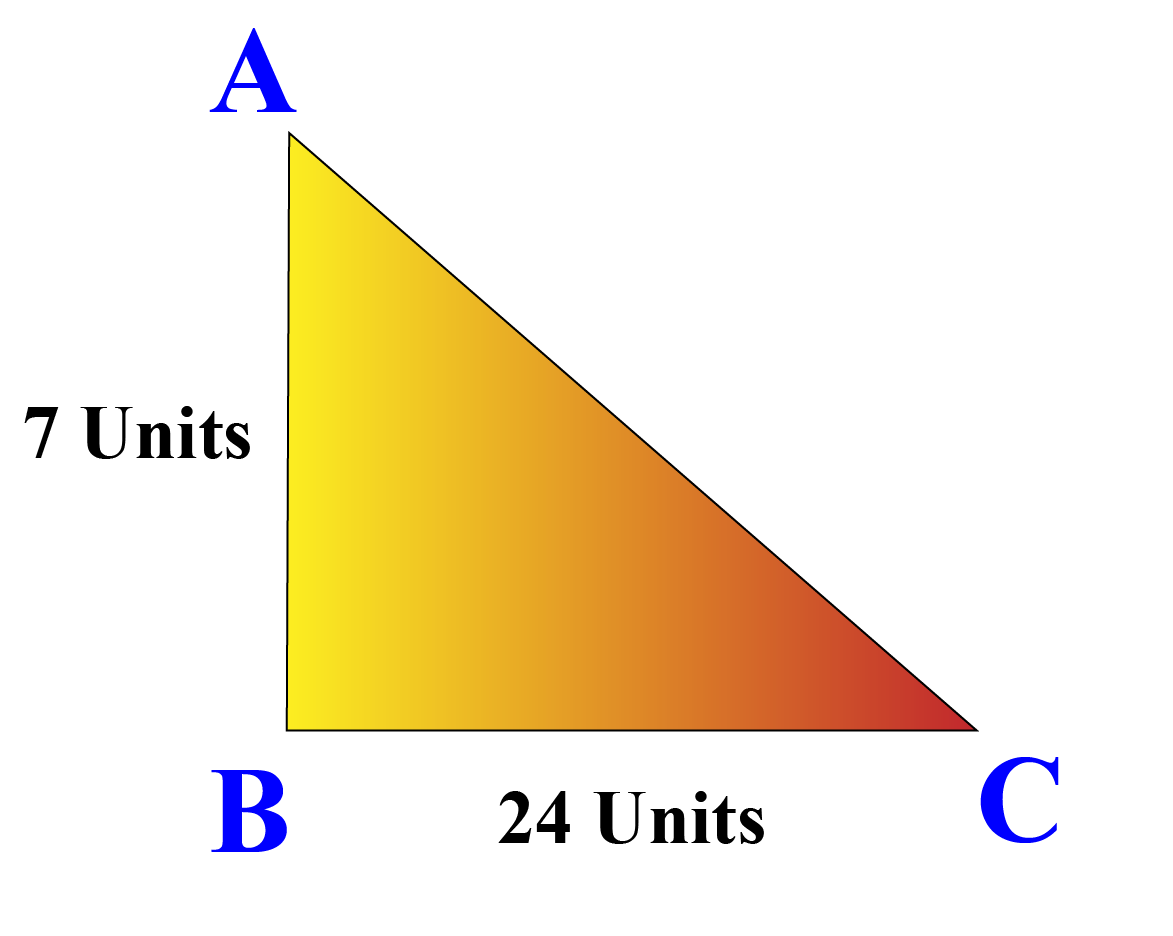# Solve for X

Judy and his friends were made to stand in rows, for a game. Judy found that if one of them is extra in a row, there would be 2 rows less and If one of them is less in a row there would be 3 rows more.

To figure out this situation he needs to learn the concept known as "Solve for x".## Lesson Plan

 1 What does solve for x mean? 2 Important Notes on Solve For x 3 Challenging Questions on Solve For x 4 Solved Examples on Solve For x 5 Interactive Questions on Solve For x

## What Does Solve for x Mean?

Solve for x means finding the value of $$x$$  for which the equation holds true. i.e when you find the value of x and substitute in the equation, we should get

LHS = RHS

If I ask you to solve the equation '$$x + 1 = 2$$' that would mean finding some value for x that satisfies the equation.

Do you think $$x=1$$ is the solution to this equation? Substitute it in the equation and see.

That’s what solving is all about.

## How Do You Solve For x?

To solve for x, bring the variable to one side, and bring all the remaining values to the other side by applying arithmetic operations on both sides of the equation.

Simplify the values to find the result.

Let’s start with simple equation as,  $$x + 2 = 7$$

How do you get x by itself?

Subtract 2 from both sides.

\begin{align} x + 2 - 2 &= 7 - 2 \\[0.2cm] x &= 5 \end{align}

Now, check the answer $$x=5$$ by substituting it back into the equation. We get 5 + 2= 7. But is this true?

Yes, so the solution (or answer) is $$x=5$$ is correct.

## Solve For x in The Triangle

"Solve for x" the unknown side or angle in a triangle we can use triangle property or the Pythagorean theorem.

Let us understand solve for x geometry by an example.

Example

$$\triangle\ ABC$$ is right-angled at $$B$$ with two of its legs measuring 7 units and  24 units. can you find the hypotenuse $$x$$?In $$\triangle ABC$$ by using the Pythagorean theorem,

we get

\begin{align} AC^2 &= AB^2 + BC^2 \\[0.2cm] x^2 &= 7^2 + 24^2 \\[0.2cm] x^2 &= 49 + 576 \\[0.2cm] x^2 &= 625 \\[0.2cm] x &= \sqrt{625} \\[0.2cm] x&=25\text{ units} \\ \end{align}

We also use properties of triangles to solve for $$x$$ in a triangle.

## Solve Equations in Different Variables

We can use a system of equations solver and solve equations with different variables.

We solve one of the equations for one variable (for example solve for x in terms of y) and then substitute it in the second equation.

We then solve this for the variable.

Finally, we substitute the value of the variable that we found in one of the equations and solve for the other variable.

Let us understand Solve for x and y by one example.

Example

Solve : $$2x - y = 5, 3x + 2y = 11$$

Solution

\begin{align} 2x - y = 5 \\ \text{Adding y on both sides we get}, \\ 2x - y + y = 5 + y \\ 2x = 5 + y \\ x = \frac{{5 + y}}{2} \\ \end{align}

Above equation is known as x in terms of y.

\begin{align} \text{Substitute x} = \frac{{5 + y}}{2}\text{in equation 2} \\ 3(\frac{{5 + y}}{2}) + 2y = 11 \\ \frac{{15 + 3y}}{2} + 2y = 11 \\ \frac{{15 + 3y + 4y}}{2} = 11 \\ \frac{{15 + 7y}}{2} = 11 \\ 15 + 7y = 22 \\ 7y = 22 - 15 \\ 7y = 7 \\ y = 1 \\ \text{Substitute y = 1 in x =} \frac{{5 + y}}{2} \\ x = \frac{{5 + 1}}{2} = \frac{6}{2} = 3 \\ \end{align}

Thus, the solution of the given system of equations is: $x=3\\y=1$

A system of two equations can be solved using different methods which you can visualize using the following algebra calculator. You can enter any two equations in two variables and choose a method. It will show the step-by-step solution in this simplify calculator.

More Important Topics
Numbers
Algebra
Geometry
Measurement
Money
Data
Trigonometry
Calculus